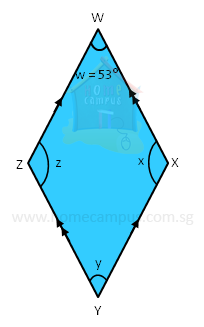## Four-sided Figures: Finding Unknown Angles - Part 1

Practice Unlimited Questions

#### 1. In the figure below, find the unknown marked angles.Since there are two pairs of parallel sides in the above figure, WXYZ is a parallelogram.

Therefore,
∠y = ∠w = 53°
Also,
∠y + ∠z = 180°
∠z = 180°  −  53° = 127°
The sum of a pair of angles between two parallel lines is 180°.
And,
∠x = ∠z = 127°
The opposite angles of a parallelogram are equal.